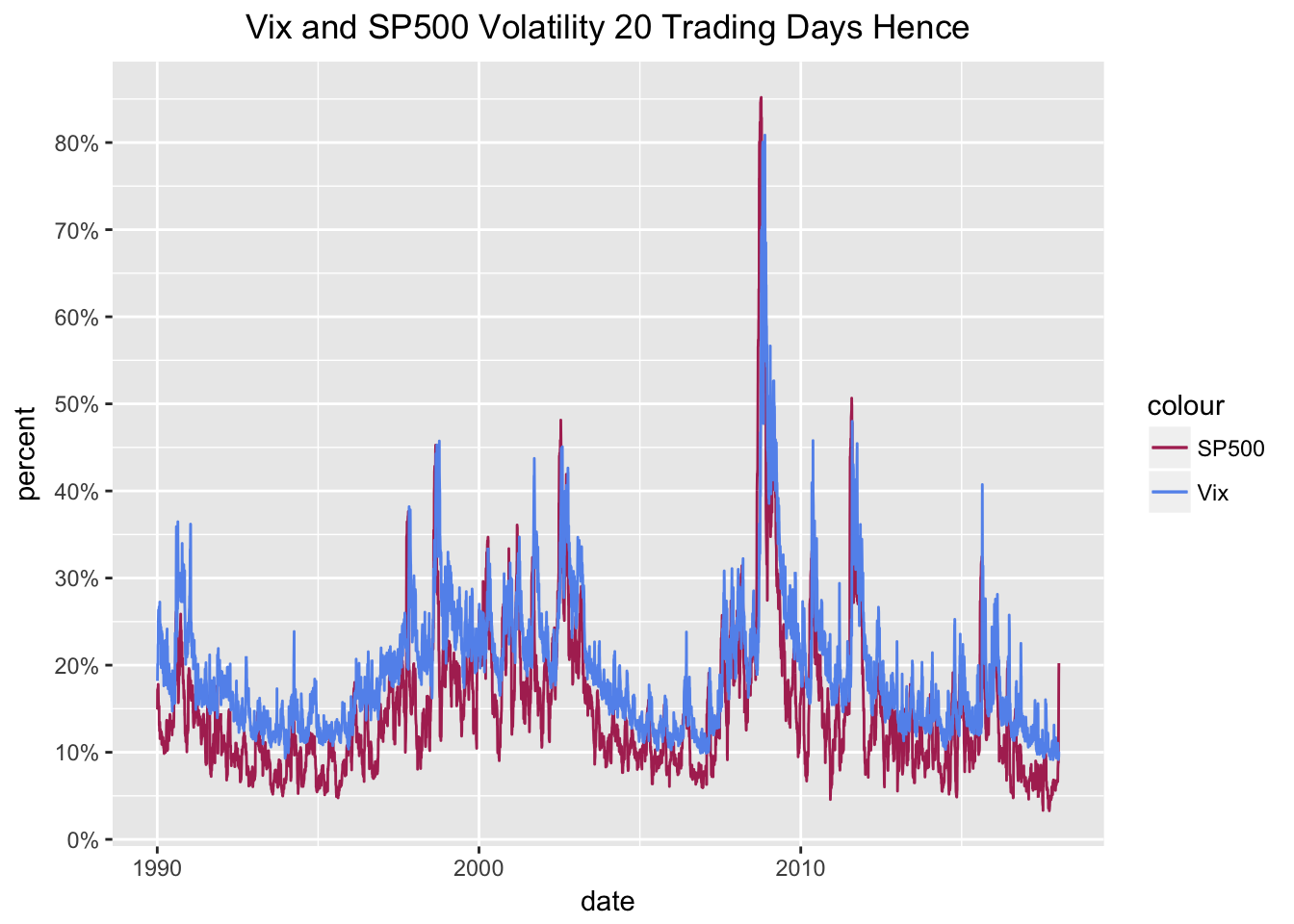# The Vix and The Future

by Jonathan Regenstein

In a previous post, we examined the relationship between the VIX and the past, realized volatility of the S&P 500.

Today, we’ll think about how/whether the VIX predicts future volatility and we’ll be reproducing some visualizations from this Bloomberg piece.

Once again, the substance and ideas here are 100% attributable to Bloomberg - my goal is to reproduce and add to our R toolkit, and learn something about volatility.

First, we grab the price history of the VIX and SP500, convert to returns, and calculate the rolling 20-day volatility of the SP500.

symbols <- c("^GSPC", "^VIX")

prices <-
getSymbols(symbols, src = 'yahoo', from = "1990-01-01",
auto.assign = TRUE, warnings = FALSE) %>%
map(~Cl(get(.)))

# get daily, cont compounded returns
sp500_returns <- na.omit(ROC(GSPC$GSPC.Close, 1, type = "continuous")) sp500_rolling_sd_20 <- rollapply(sp500_returns, 20, function(x) StdDev(x)) sp500_rolling_sd_annualized_20 <- (round((sqrt(252) * sp500_rolling_sd_20 * 100), 2)) vol_vix_xts <- merge.xts(VIX$VIX.Close, sp500_rolling_sd_annualized_20)

We have VIX prices and rolling 20-day SP500 volatility. We want to reproduce the visualizations that illuminate how the VIX predicts realized volatility. In other words, is the VIX reading on 2017-01-01 telling us anything about realized volatility 20 days from 2017-01-01?

The data wrangling challenge here is all about the dates. We need to compare the VIX reading on 2017-01-01 to the realized volatility that occurs over the 20 days after 2017-01-01. It’s not hard to understand this in our heads, it’s a little tricky to line the data up correctly (full disclosure: I had to go to a whiteboard and draw out 20 cells with arrows for what need to go where from the data frame.)

First, we’ll set a start date as the first date index of sp500_rolling_sd_annualized_20 and an end date for the date index that is 19 days before end of sp500_rolling_sd_annualized_20.

start.date <- index(sp500_rolling_sd_annualized_20)

end.date <- index(sp500_rolling_sd_annualized_20[nrow(sp500_rolling_sd_annualized_20) - 19])

OK, we will start on 1990-01-03 and end on 2018-01-09.

Now let’s grab the SP500 rolling volatility data between those dates. Note we use the coredata() function because we don’t need the time index, just the data.

# Extract the data, not the time index since we're adding to a tibble.
future_sp500_vol <- tibble::as_tibble   (coredata(sp500_rolling_sd_annualized_20[20:nrow(sp500_rolling_sd_annualized_20), ]))

Now we want to get the VIX data for those same dates and combine them into one tibble. We will get the VIX data with VIX$VIX.Close[paste(start.date, end.date, sep = "::")]. vix_sp500_future_vol <- # Get VIX data VIX$VIX.Close[paste(start.date, end.date, sep = "::")] %>%
tk_tbl(preserve_index = TRUE, rename_index = "date") %>%
select(date, everything()) %>%
# Add the SP500 data with bind_cols - important line of code here!
bind_cols(future_sp500_vol) %>%
rename(vix = VIX.Close, Spy.future = GSPC.Close)

tail(vix_sp500_future_vol)
## # A tibble: 6 x 3
##   date         vix Spy.future
##   <date>     <dbl>      <dbl>
## 1 2018-01-02  9.77       9.10
## 2 2018-01-03  9.15       8.88
## 3 2018-01-04  9.22       8.81
## 4 2018-01-05  9.22      12.1
## 5 2018-01-08  9.52      19.1
## 6 2018-01-09 10.1       20.2

Take a peek at the result. We now have a tibble with a column for the VIX price on date t, and a column for realized, future volatility 20 days after date t.

Now it’s on to ggplot to recreate the visualizations from Bloomberg.

line_future_vol20 <-
# Plot vix on the x axis and future vol on the y axis
ggplot(vix_sp500_future_vol, aes(date)) +
geom_line(aes(y = Spy.future, colour = "SP500")) +
geom_line(aes(y = vix, colour = "Vix")) +
scale_color_manual(values = c(SP500 = 'maroon', Vix = 'cornflowerblue')) +
# The remaining lines are aesthetic, title, axis labels.
ggtitle("Vix and SP500 Volatility 20 Trading Days Hence") +
xlab("date") +
ylab("percent") +
scale_y_continuous(labels = function(x){ paste0(x, "%") },
breaks = scales::pretty_breaks(n=10)) +
theme(plot.title = element_text(hjust = 0.5))

line_future_vol20I can’t eyeball much from that chart so let’s move to a scatter plot. We want to have a different color and shape scheme for different dates so we’ll use nested if/else statements to create three eras: pre-historic, Obama, Trump.

vix_sp500_future_vol$era <- ifelse(vix_sp500_future_vol$date <= "2008-11-03", 'pre-historic',
ifelse(vix_sp500_future_vol$date >"2008-11-04" & vix_sp500_future_vol$date <=  "2016-11-08", 'Obama', 'Trump'))

By creating that era column, we can pass it to aes() for a nicer chart and legend.

scatter_future_vol20 <-
ggplot(vix_sp500_future_vol, aes(vix, Spy.future, color = era, shape = era)) +
geom_point() +
scale_color_manual(values=c("maroon", "cornflowerblue", "green")) +
scale_shape_manual(values = c(17, 15, 18)) +
geom_line(aes(vix, vix), color = "black") +
# The remaining lines are aesthetic, title, axis labels.
ggtitle("Vix v. Subsequent Volatility") +
xlab("Vix") +
ylab("Realized vol 20 subsequent trading days") +
scale_y_continuous(labels = function(x){ paste0(x, "%") },
breaks = scales::pretty_breaks(n=10)) +
scale_x_continuous(labels = function(x){ paste0(x, "%") },
breaks = scales::pretty_breaks(n=10)) +
theme(plot.title = element_text(hjust = 0.5))

scatter_future_vol20That diagonal black line is the volatility predicted by the VIX and we can see that actual volatility tends to be a bit lower than predicted. The maroon diamonds are the observations during the Obama administration. The green triangles are the observations since November 8, 2016. Over the last ~9 years, realized volatility has generally been lower than what the VIX was predicting. Indeed, it’s hard to look at those green diamonds and worry too much about a volatility spike right now.

Another interesting note: realized one-month volatility has not reached 20% when the VIX was below 12% (add a vertical line at 12% to see this more clearly). As long as the VIX stays below 12%, it’s hard to get too worried about subsequent volatility.

For the well-articulated insights and theory, have a look at the original piece on Bloomberg.